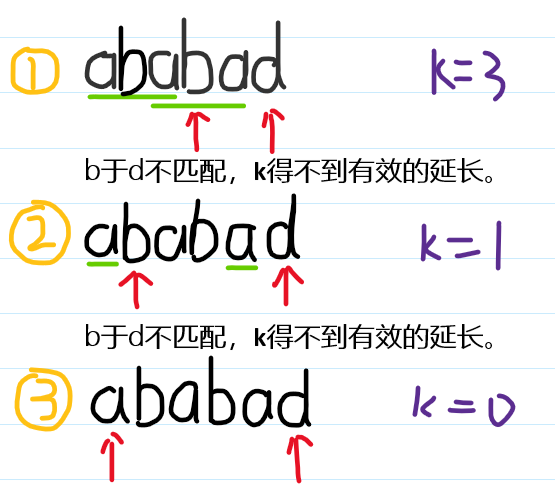# 字符串&数组

## 17.电话号码的字母组合

``````class Solution:
def letterCombinations(self, digits: str) -> List[str]:
map={'2':"abc",'3':"def",'4':"ghi",'5':"jkl",'6':"mno",'7':"pqrs",'8':"tuv",'9':"wxyz"}
if not digits: return []
ans=[""]
for i in digits:
ans=[pre+suf for pre in ans for suf in map[i]]
return ans``````

## 696.计数二进制子串

``````class Solution:
def countBinarySubstrings(self, s: str) -> int:
temp=
ans=0
for i in range(1,len(s)):
if s[i]==s[i-1]:
temp[-1]+=1
else:
temp.append(1)
for i in range(len(temp)-1):
ans+=min(temp[i],temp[i+1])
return ans

# 计算相邻数的频数
# 看着有些抽象``````

## 605.种花问题

``````class Solution:
def canPlaceFlowers(self, f: List[int], n: int) -> bool:
f=+f+[0,1]
ans,cnt=0,0
for i in f:
if i==0: cnt+=1
else:
ans+=(cnt-1)//2
cnt=0
#print(ans,n)
return ans>=n

# 数组，两端加值便于解题。``````

## 75.颜色分类

``````#荷兰国旗问题，快速排序基础
class Solution:
def sortColors(self, nums: List[int]) -> None:
i,l,r=0,0,len(nums)-1
while i<=r:
if nums[i]==0:
nums[i],nums[l]=nums[l],nums[i]
l+=1
i+=1
elif nums[i]==2:
nums[i],nums[r]=nums[r],nums[i]
r-=1
else: i+=1``````

## 74.搜索二维矩阵

• 每行中的整数从左到右按升序排列。
• 每行的第一个整数大于前一行的最后一个整数。
``````class Solution:
def searchMatrix(self, matrix: List[List[int]], target: int) -> bool:
rows,cols=len(matrix),len(matrix)
l,r=0,rows*cols-1
while l<=r:
mid=(l+r)//2
x,y=mid//cols,mid%cols
if matrix[x][y]==target: return True
elif matrix[x][y]>target: r=mid-1
else: l=mid+1
return False

#原地二分查找``````

## 852.山脉数组的峰顶索引

``````class Solution:
def peakIndexInMountainArray(self, arr: List[int]) -> int:
l, r = 0, len(arr)-1
while l<r:
mid = (l+r)//2
if arr[mid] > arr[mid+1]:
r = mid
else:
l = mid + 1
return l

#二分的变式``````

## kmp算法

kmp是一个效率非常高的字符串匹配算法。

``````#求b在a中出现次数
a = "ababacababadababadadda"
b = "ababad"``````

kmp可以将暴力法的`O(m*n)`降低为`O(m+n)`

1. 计算temp数组
temp数组可理解为一组b中相同前后缀的标记(不能为本身长度)

`b = "ababad"`

``````def cal_temp(b):
#K是一个对相同前后缀的标记
temp,k=,0
#从索引1处开始遍历
for i in range(1,len(b)):
while k>0 and b[i]!=b[k]:
k=temp[k-1]
if b[i]==b[k]:
k+=1
temp.append(k)
return temp``````

• `i=1`时，’ab’，b!=b，temp.append(0)
• `i=2`时，’aba’，b==b，temp.append(1)
• `i=3`时，’abab’，b==b，temp.append(2)
• `i=4`时，’ababa’，b==b，temp.append(3)
• `i=5`时，’ababad’，temp=[0,0,1,2,3]
b!=b，k=temp[3-1]=1
b!=b，k=temp[1-1]=0
temp.append(0)

`i=5`时，’ababad’，temp=[0,0,1,2,3]，k=3aba and aba can match,k=3
a and a can match,k=(aba的匹配数1,即temp[k-1])
more explain: aba can see as a and a,the first `a` can match the fourth `a`

2. kmp

``````def kmp(a,b):
temp=cal_temp(b)
ans,k=0,0
for i in range(len(a)):
while k>0 and a[i]!=b[k]:
k=temp[k-1]
if a[i]==b[k]:
k+=1
if k==len(b):
ans+=1
k=temp[k-1]
return ａｎｓ

#小结：利用已匹配的信息，迈出比较大的步子。``````

目录